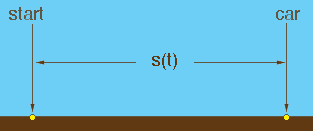SEARCH HOMEMath Central Quandaries & QueriesQuestion from Gerry: In mathematical context, what do you understand by the term "Second Derivative"Hi Gerry,

This is probably most easily understood with an example.

Suppose you are on a straight road travelling in a car. Suppose also that you started from some particular place on the road and at the moment you started moving you also started a stopwatch. I am going to let s(t) be the distance between you and the start point, in kilometers, at the time when the stopwatch reads t hours. The illustration below represents the situation at time t hours.There is a mathematical process called differentiation that derives from the function s(t) another function called the derivative of s(t) or sometimes the first derivative of s(t). This new function is usually written s'(t) or ds/dt. s'(t) measures the rate at which the function s(t) is changing at the instant when the time is t hours. If the car is moving away from the start then s'(t) is positive. If the car is moving towards the start then s(t) is decreasing and s'(t) is negative. If the car is stopped then s'(t) = 0. This function s'(t) is the speed or velocity of the car and is approximated by the speedometer in the car. The units of s'(t) are kilometers/hour.

If the car speeds up then the velocity increases, that is s'(t) increases. Hence s'(t) is changing and we have seen how to measure the rate at which a function is changing using its derivative. Thus s''(t), the derivative of s'(t), measures the rate at which the velocity is changing. s''(t) is the second derivative of s(t). s''(t) is the acceleration of the car. If the velocity is increasing then s''(t) is positive. If the velocity is decreasing, the car is slowing down, then s''(t) is negative. If the car is travelling at a constant speed then s''(t) = 0. The units of s''(t) are kilometers/hour2.

For any function f(x) the first derivative f'(x) measures the rate at which f(x) is changing relative to x and the second derivative f''(x) measures the rate at which f'(x) is changing.

I hope this helps,
PennyMath Central is supported by the University of Regina and The Pacific Institute for the Mathematical Sciences.